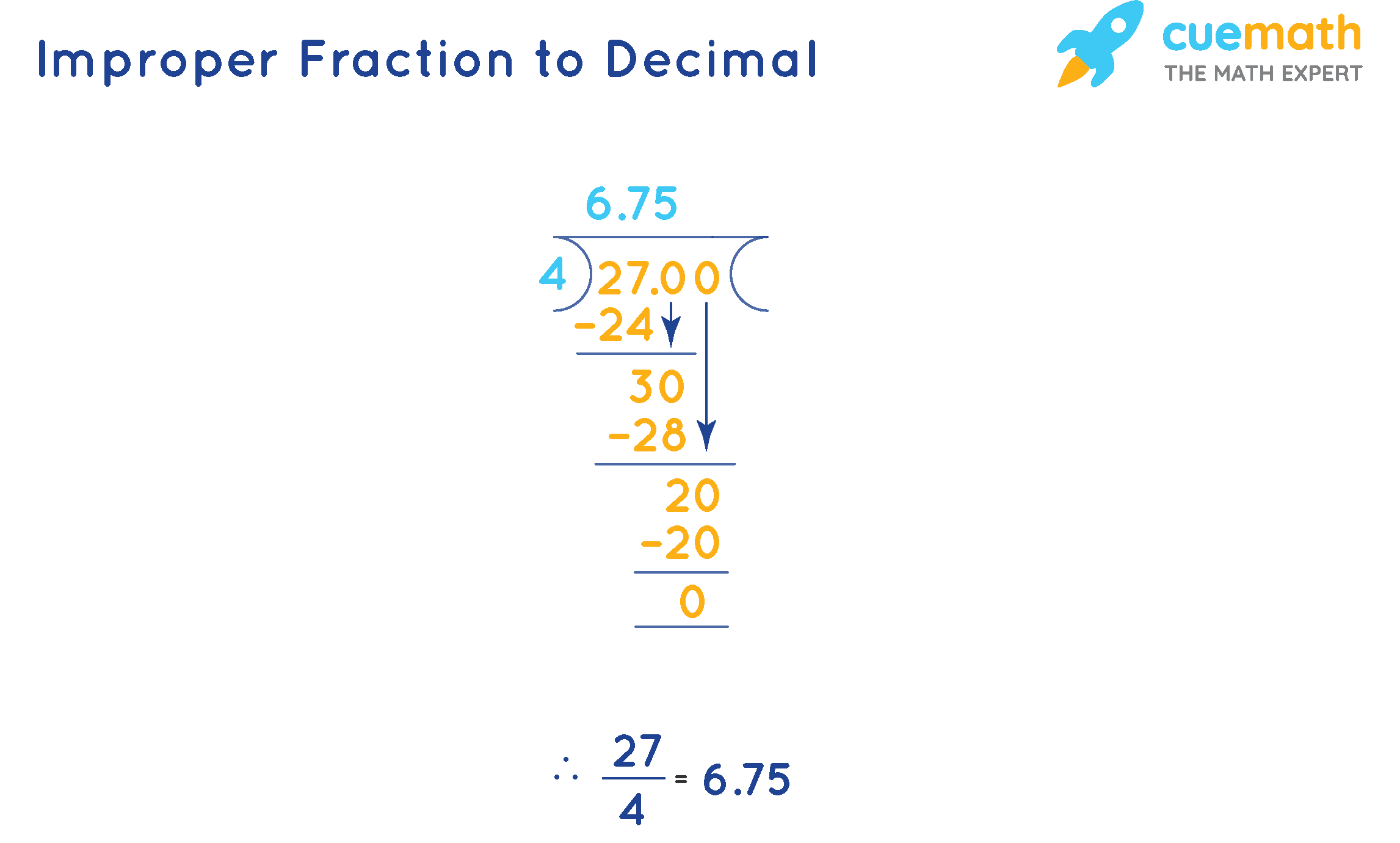# Mixed Number to Decimal Calculator

## What Is a Mixed Number to Decimal Calculator?

A Mixed Number to Decimal Calculator is a free online tool which converts a mixed fraction to a decimal number. Cuemath's online calculator helps you to calculate faster and converts mixed numbers to decimal numbers within a few seconds.

Note: Enter numbers up to 2 digits.

## How to Use the Mixed Number to Decimal Calculator?

Follow the steps given below to use the calculator:

• Step 1: Enter the mixed fraction in the input box.
• Step 2: Click on "Calculate" to get the decimal number for the mixed fraction that was entered.
• Step 3: Click on "Reset" to clear the fields and enter a new fraction.

## How to Convert a Mixed Fraction to a Decimal Number?

A mixed fraction or a mixed number is the combination of a whole number and a proper fraction. A mixed fraction can be represented as a decimal number in the following way:

• Convert the mixed fraction to an improper fraction.
• Now, convert the improper fraction to a decimal number by dividing the numerator by the denominator.

### Solved Example:

Convert $$6\frac{3}{4}$$ to a decimal number.

### Solution:

$$6\frac{3}{4}$$  can be converted to an improper fraction as 27/4

Now, divide 27 by 4.Therefore, $$6\frac{3}{4}$$, in its decimal form is 6.75

Now, try to use this calculator and convert the following mixed fractions to decimal numbers:

%--split--%